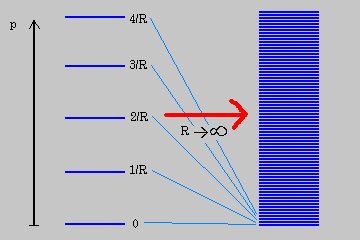## Tuesday, January 24, 2006

### Spacetime 101Here's some basic background covering how mathematical models of space and time have evolved since ancient times, from the Pythagorean Rule to Newtonian mechanics, Special Relativity and General Relativity.For the roads leading to one's view of the strange world of non-euclidean views had to offer, I of course needed some model from which to work. As I looked at the model above and the transfer of higher dimensional thinking, the very idea and contrast to the lower image represented, how would you associate gravity in the diagram but watch the circle valution along side of gravity that emegres from the 2d discription as a energy valution, and relationship to gravity, evolving from mass, energy interconnectivity. I have to apologize as I was developing and am developing.I do not know if this is right to assign my view above, while one did not know the evaluation of 1R as I watch DRL assessment of what can no longer be considered as valid, I have to wonder why such observations are not thought about more intricately as the valuation of that circle is considered. The comparison was drawn between the two pictures of the spacetime fabric above here, and below.Let's now start analysing a 2D case, that of the classic Flatland example, in which a person lives in a 2D universe and is only aware of two dimensions (shown as the blue grid), or plane, say in the x and y direction. Such a person can never conceive the meaning of height in the z direction, he cannot look up or down, and can see other 2D persons as shapes on the flat surface he lives in.

So if you follow the dimensional analysis, there is a systemic procedure that one has to follow, that does not have to be held in context of KK interpretation to this point, but it does help if you think about the very basis of this graduation that certain statements make themself known.

Degrees of freedom(Wiki 24 Jan 2006)

Zero dimensions
Point
Zero-dimensional space
One dimension
Line
Two dimensions
2D geometric models
2D computer graphics
Three dimensions
3D computer graphics
3-D films and video
Stereoscopy (3-D imaging)
Four dimensions
Time (4th dimension)
Fourth spatial dimension
Tesseract (four dimensional shapes)
Five dimensions
Kaluza-Klein theory
Fifth dimension
Ten, eleven or twenty-six dimensions
String theory
M-theory
Why 10 dimensions?
Calabi-Yau spaces
Infinitely many dimensions
Banach space (only some have infinitely many dimensions)
Special relativity
General relativity

Would you dimiss a comment by Greene because of the speculation you have felt about him that you might not recognize, what is being said as you watch that circle develope alongside of the sphere, as it moves through the 2d discription? Here's what mean, as I had focused on Brian Greene's words.Angular momentum can twist light cones and even make time travel possible in theory if not in practice.

The familiar extended dimensions, therefore, may very well also be in the shape of circles and hence subject to the R and 1/R physical identification of string theory. To put some rough numbers in, if the familiar dimensions are circular then their radii must be about as large as 15 billion light-years, which is about ten trillion trillion trillion trillion trillion (R= 1061) times the Planck length, and growing as the universe explands. If string theory is right, this is physically identical to the familiar dimensions being circular with incredibly tiny radii of about 1/R=1/1061=10-61 times the Planck length! There are our well-known familiar dimensions in an alternate description provided by string theory. [Greene's emphasis]. In fact, in the reciprocal language, these tiny circles are getting ever smaller as time goes by, since as R grows, 1/R shrinks. Now we seem to have really gone off the deep end. How can this possibly be true? How can a six-foot tall human being 'fit' inside such an unbelievably microscopic universe? How can a speck of a universe be physically identical to the great expanse we view in the heavens above?
( Brian Greene, The Elegant Universe, pages 248-249)

Fifth dimension(wiki 24 Jan 2006)
Abstract, five dimensional space occurs frequently in mathematics, and is a perfectly legitimate construct. Whether or not the real universe in which we live is somehow five-dimensional is a topic that is debated and explored in several branches of physics, including astrophysics and particle physics.

Five dimensions in physics
(Wiki 24 Jan 2006)

In physics, the fifth dimension is a hypothetical dimension which would exist at a right angle to the fourth dimension